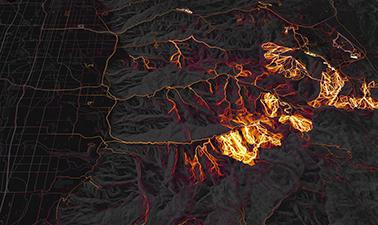# Data Science: Machine Learning and Predictions

MOOCEnglish1 hour coourseCertification paid
Course by EdX
freefree
What will you learn?
Fundamental concepts of machine learning
Linear regression, correlation, and the phenomenon of regression to the mean
Classification using the k-nearest neighbors algorithm
How to compare and evaluate the accuracy of machine learning models
Basic probability and Bayes’ theorem

One of the principal responsibilities of a data scientist is to make reliable predictions based on data. When the amount of data available is enormous, it helps if some of the analysis can be automated. Machine learning is a way of identifying patterns in data and using them to automatically make predictions or decisions. In this data science course, you will learn basic concepts and elements of machine learning.

The two main methods of machine learning you will focus on are regression and classification. Regression is used when you seek to predict a numerical quantity. Classification is used when you try to predict a category (e.g., given information about a financial transaction, predict whether it is fraudulent or legitimate).

For regression, you will learn how to measure the correlation between two variables and compute a best-fit line for making predictions when the underlying relationship is linear. The course will also teach you how to quantify the uncertainty in your prediction using the bootstrap method. These techniques will be motivated by a wide range of examples.

For classification, you will learn the k-nearest neighbor classification algorithm, learn how to measure the effectiveness of your classifier, and apply it to real-world tasks including medical diagnoses and predicting genres of movies.

The course will highlight the assumptions underlying the techniques, and will provide ways to assess whether those assumptions are good. It will also point out pitfalls that lead to overly optimistic or inaccurate predictions.

Program
Foundations of Data Science: Prediction and Machine Learning
Learn how to use machine learning, with a focus on regression and classification, to automatically identify patterns in your data and make better predictions.
Lecturers